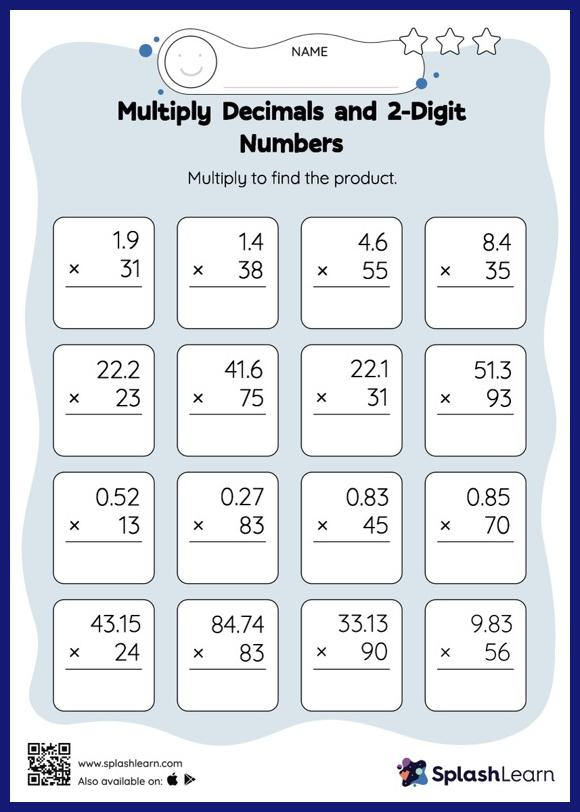# Multiply Decimals and 2-Digit Numbers: Vertical Multiplication Worksheet

Home > Multiply Decimals and 2-Digit Numbers: Vertical MultiplicationMultiply decimals and 2-digit numbers worksheet helps students develop proficiency with multiplication. When multiplying a decimal by a two-digit number, students may ignore the decimal point during calculations and bring it back when writing the result. This multiply decimals and 2-digit numbers worksheet provides plenty of practice with this concept. In each problem, the numbers are laid out in the vertical format. In this format, pair of digits in each successive place are tackled one by one. This helps students follow a structured approach.